## Steiner Chain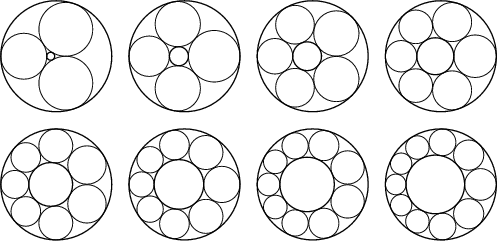Given two nonconcentric Circles with one interior to the other, if small Tangent Circles can be inscribed around the region between the two Circles such that the final Circle is Tangent to the first, the Circles form a Steiner chain.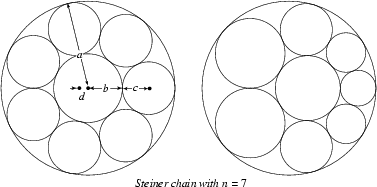The simplest way to construct a Steiner chain is to perform an Inversion on a symmetrical arrangement oncircles packed between a central circle of radiusand an outer concentric circle of radius(Wells 1991). In this arrangement,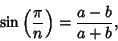(1)

so the ratio of the radii for the small and large circles is(2)

To transform the symmetrical arrangement into a Steiner chain, take an Inversion Center which is a distancefrom the center of the symmetrical figure. Then the radiiandof the outer and center circles become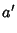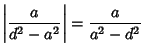(3)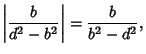(4)

respectively. Equivalently, a Steiner chain results whenever the Inversive Distance between the two original circles is given by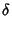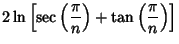(5)(6)

(Coxeter and Greitzer 1967).

Steiner's Porism states that if a Steiner chain is formed from one starting circle, then a Steiner chain is also formed from any other starting circle.

See also Arbelos, Coxeter's Loxodromic Sequence of Tangent Circles, Hexlet, Pappus Chain, Steiner's Porism

References

Coxeter, H. S. M. Interlocking Rings of Spheres.'' Scripta Math. 18, 113-121, 1952.

Coxeter, H. S. M. Introduction to Geometry, 2nd ed. New York: Wiley, p. 87, 1969.

Coxeter, H. S. M. and Greitzer, S. L. Geometry Revisited. Washington, DC: Math. Assoc. Amer., pp. 124-126, 1967.

Forder, H. G. Geometry, 2nd ed. London: Hutchinson's University Library, p. 23, 1960.

Gardner, M. Mathematical Games: The Diverse Pleasures of Circles that Are Tangent to One Another.'' Sci. Amer. 240, 18-28, Jan. 1979.

Johnson, R. A. Modern Geometry: An Elementary Treatise on the Geometry of the Triangle and the Circle. Boston, MA: Houghton Mifflin, pp. 113-115, 1929.

Ogilvy, C. S. Excursions in Geometry. New York: Dover, pp. 51-54, 1990.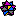Weisstein, E. W. Plane Geometry.'' Mathematica notebook PlaneGeometry.m.